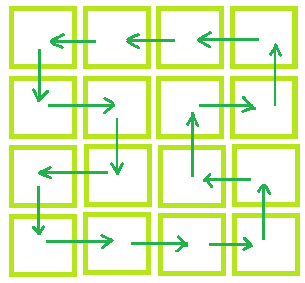Check if possible to shuffle a matrix with adjacent movements

• Last Updated : 16 Apr, 2021

Given a N x M matrix which represents the number of rows and number of columns respectively. Each cell of a matrix is occupied by exactly one student. The task is to determine whether we can shuffle each student in such a way that each student should occupy a cell that is adjacent to that student’s original cell, i.e. immediately to the left, right, top or bottom of that cell and after shuffling each cell should occupy by exactly one student.
Examples:

Input: N = 3, M = 3
Output: Shuffling not possible
Input: N = 4, M = 4
Output: Shuffing is possible
One possible way to shuffle the student is as shown below:Approach: Check if the number of rows or the number of columns is even then shuffling is possible otherwise no shuffling is possible.
Below is the implementation of the above approach:

C++

 // C++ implementation of above approach #include using namespace std;   // Function that will check whether // Shuffling is possible or not void Shuffling(int N, int M) {     if (N % 2 == 0 || M % 2 == 0)         cout << "Shuffling is possible";     else         cout << "Shuffling not possible"; }   // Driver code int main() {     int N = 4, M = 5;       // Calling function.     Shuffling(N, M);     return 0; }

Java

// Java implementation of above approach
import java.io.*;

class GFG
{

// Function that will check whether
// Shuffling is possible or not
static void Shuffling(int N, int M)
{
if (N % 2 == 0 || M % 2 == 0)
System.out.println("Shuffling is possible");
else
System.out.println("Shuffling not possible");
}

// Driver code
public static void main (String[] args)
{
int N = 4, M = 5;

// Calling function.
Shuffling(N, M);
}
}

// This code is contributed by anuj_67..

Python3

 # Python3 implementation of above approach   # Function that will check whether # Shuffling is possible or not def Shuffling(N, M) :           if (N % 2 == 0 or M % 2 == 0) :         print("Shuffling is possible");     else :         print("Shuffling not possible");   # Driver Code if __name__ == "__main__" :       # Driver code     N = 4;     M = 5;       # Calling function.     Shuffling(N, M);       # This code is contributed by Ryuga

C#

 // C# implementation of above approach using System;   class GFG {       // Function that will check whether // Shuffling is possible or not static void Shuffling(int N, int M) {     if (N % 2 == 0 || M % 2 == 0)         Console.Write("Shuffling is possible");     else         Console.Write("Shuffling not possible"); }       // Driver code     public static void Main ()     {         int N = 4, M = 5;           // Calling function.         Shuffling(N, M);     } }   // This code is contributed by Ita_c.



Javascript



Output:

Shuffling is possible

Want to learn from the best curated videos and practice problems, check out the C++ Foundation Course for Basic to Advanced C++ and C++ STL Course for the language and STL. To complete your preparation from learning a language to DS Algo and many more, please refer Complete Interview Preparation Course.

My Personal Notes arrow_drop_up
Recommended Articles
Page :